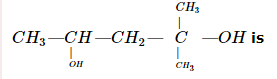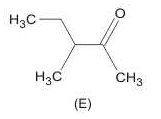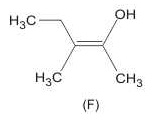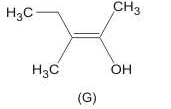Courses

# WBJEE Chemistry Test - 15

## 40 Questions MCQ Test WBJEE Sample Papers, Section Wise & Full Mock Tests | WBJEE Chemistry Test - 15

Description
This mock test of WBJEE Chemistry Test - 15 for JEE helps you for every JEE entrance exam. This contains 40 Multiple Choice Questions for JEE WBJEE Chemistry Test - 15 (mcq) to study with solutions a complete question bank. The solved questions answers in this WBJEE Chemistry Test - 15 quiz give you a good mix of easy questions and tough questions. JEE students definitely take this WBJEE Chemistry Test - 15 exercise for a better result in the exam. You can find other WBJEE Chemistry Test - 15 extra questions, long questions & short questions for JEE on EduRev as well by searching above.
QUESTION: 1

Solution:
QUESTION: 2

Solution:
QUESTION: 3

### Treatment of furfural with NaOH yields

Solution:
QUESTION: 4

The energy of an orbit in a hydrogen atom is given by the relation
E = -(Constant/n2) kJ mol-1.
Which of the following properties represents the constant in the above relation?

Solution:
QUESTION: 5
When AgNO₃ is strongly heated, the products formed are
Solution:
QUESTION: 6
Which of the following is not present in RNA?
Solution:
QUESTION: 7

A light greenish coloured salt was soluble in water. On passing H₂S into the solution, a black ppt. was obtained which dissolved readily in HCl. The metal ion present is

Solution:
QUESTION: 8
A $\sigma$- bond is
Solution:
QUESTION: 9

The state of hybridisation of boron and oxygen atoms in boric acid molecule (H₃BO₃) are respectively

Solution:
QUESTION: 10

If bond enthalpies of H-H,Br-Br and H-Br bonds are 433 ,192 and 364 KJ mol-1 respectively ,The ∆Hfor the reaction H₂(g) + Br₂(g) →2HBr(g) is

Solution:
QUESTION: 11
Vanishing creams differ from other creams due to the presence of
Solution:
QUESTION: 12
The equilibrium constant in a reversible chemical reaction at a given temperature
Solution:
QUESTION: 13
A reaction involving two different reactants can never be
Solution:
QUESTION: 14
For a system in equilibrium, ∆G=0, under conditions of constant
Solution:
QUESTION: 15
The number and type of bonds between two carbon atoms in calcium carbide are
Solution:
QUESTION: 16

The paramagnetic cation is

Solution:
QUESTION: 17
The right order of the solubility of sulphates of alkaline earth metals in water is
Solution:
QUESTION: 18

3.2 g of oxygen (At wt. = 16) and 0.2 g of hydrogen (At. wt. = 1) are placed in a 1.12 litre flask at 0oC. The total pressure of the gas mixture will be

Solution:
QUESTION: 19
Orthophosphoric acid is
Solution:
QUESTION: 20
Which metal is protected by a layer of its own oxide?
Solution:
QUESTION: 21
Optical glass contains a high precentage of
Solution:
QUESTION: 22
Hydrogen from HCl can be prepared by
Solution:
QUESTION: 23
Which of the following compound is optically inactive?
Solution:
QUESTION: 24
When a slow neutron goes sufficently close to a U-235 nucleus, then the process which takes place is called
Solution:
QUESTION: 25
A Beckmann rearrangement is affected by
Solution:
QUESTION: 26
Synthetic polymer which resemble natural rubber is
Solution:
QUESTION: 27
A, B, C are three element forming a part of compound in oxidation states of +2, +5 and -2 respectively. What could be the compound?
Solution:
QUESTION: 28
Copolymer is
Solution:
QUESTION: 29
The interionic distance for cesium chloride cystal will be
Solution:
QUESTION: 30
Consider the following statements about dimethyl cyclohexanes :
1. trans-1,2-dimethylcyclohexane is more stable than the corresponding cis-isomer
2. cis-1,3-dimethylcyclohexane is an optically inactive meso form
3. trans-1,3-dimethylcyclohexane is more stable than the corresponding cis-isomer
4. cis-1,2-dimethylcyclohexane is an unresolvable racemic mixture
Which of the statements given above are correct ?
Solution:
QUESTION: 31

In the following question, a Statement of Assertion (A) is given followed by a corresponding Reason (R) just below it. Read the Statements carefully and mark the correct answer-

Assertion(A): According to VSEPR theory, there shall be minimum repulsions among lone pairs and bond pairs present in a molecule or ion.

Reason(R): I3- , has a linear structure.

Solution:
QUESTION: 32

The hydrocarbon which can react with sodium in liquid ammonia is

Solution:
QUESTION: 33

Toluene is nitrated and the resulting product is reduced with tin and hydrochloric acid . The product so obtained is diazotised and then heated with cuprous bromide. Thr reaction mixture so formed contains

Solution:
QUESTION: 34

The half-life period of a first order chemical reaction is 6.93 minutes. The time required for the completion of 99% of the chemical reaction will be (log 2 = 0.301).

Solution:
QUESTION: 35

The IUPAC name of the compoundSolution:
QUESTION: 36

Which are ionic compounds?

Solution:
QUESTION: 37

The reagents , NH4Cl and aqueous NH3 will precipitate :

Solution:
QUESTION: 38

The correct statement(s) concerning the structures E, F and G is (are)Solution:
QUESTION: 39

Which of the following alloys contain(s) Cu and Zn ?

Solution:
QUESTION: 40

Identify the correct statement of the followings?

Solution: